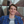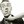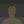0%
0%

# ESP32 as BT receiver with DSP-capabilities

Similar projects worth following
10.4k views
I'm trying to build an advanced BT receiver for mobile speakers based on the ESP32 and a PCM5102 DAC. So far biquad filters and a startup sound are implemented.

Code-base is espressif's a2dp_sink example: https://github.com/espressif/esp-idf/tree/master/examples/bluetooth/a2dp_sink

Unfortunately I still did not have much time to document the project properly. However if you are a bit familiar with the ESP32 and ESP-IDF, you should get along with the instructions :)

• 1 × ESP32 Microcontroller
• 1 × PCM5102 Data Converters / Digital to Analog Converters (DACs)

• ### ToDo

Raphael H06/22/2019 at 20:06 0 comments

- Better/more detailed instructions

- Audio processing for startup sound

- More efficient way to store startup-sound (mono, maybe lower samplerate?, maybe only 8Bit?)

- Other functions for a mobile speaker, e.g. battery watchdog

• 1
Setting up the hardware

To build your own bluetooth-receiver you only need an ESP32 and a PCM5102 DAC.

I soldered the DAC directly to the ESP32 but you could also use a breakout board. The a2dp_sink uses the following pins at default:

 ESP Pin I2S Signal GPIO22 LRCK GPIO25 DATA GPIO26 BCK

However I changed the setup the following, since I will solder the DAC directly to the ESP32:

 ESP Pin I2S Signal PCM5102 Pin GPIO15 LRCK LRCK GPIO2 DATA DIN GPIO4 BCK BCK GND GND 3V3 VIN

Now you can remove the RX2 Pin from the ESP32 since we wont give the DAC a SCK. It will generate this clock from the other clocks we are providing. I put a little tape strip over the connection to prevent it from short circuiting if the pin is used for other stuff. You also have to solder the sck bridge on the front of the DAC. Afterwards you can put the DAC directly under the ESP32 like this:

Now you can solder the pins together:

Now the hardware is prepared succesfully.

• 2
Setting up your project on the PC

First you need to install the ESP-IDF as described here: https://docs.espressif.com/projects/esp-idf/en/latest/get-started/

Afterwards you can copy the folder \examples\bluetooth\a2dp_sink\ to your project home directory. Change your path in msys to the copied folder using "cd .....". With the command "make menuconfig" you can start the setup. Here you have to change the pins in the a2dp_sink expample configuration to the pins you connected to the DAC:

You also have to set the right COM port in the serial flasher configuration. Safe the configuration and exit the menuconfig.

Afterwards you can connect the ESP32 to your PC and test if everything is working. To try this you have to use the command "make flash". After the flashing is competed you can find a a2dp sink bluetooth device. After you paired our device to it you can start playing some audio, you should hear it if you connect your headphones to the DAC.

• 3

Open main\bt_app_av.c

Replace the function void bt_app_a2d_data_cb(const uint8_t *data, uint32_t len) with the following code:

```void bt_app_a2d_data_cb(const uint8_t *data, uint32_t len)
{
size_t bytes_written;
uint8_t *mydat = malloc(len);
if(mydat == NULL)
{
ESP_LOGI(BT_AV_TAG, "Not enough memory available");
}
else
{
for (uint32_t i = 0; i < len; i+=4)
{
sample_l_int = (int16_t)((*(data + i + 1) << 8) | *(data + i));
sample_r_int = (int16_t)((*(data + i + 3) << 8) | *(data + i +2));
sample_l_float = (float)sample_l_int / 0x8000;
sample_r_float = (float)sample_r_float / 0x8000;
in = (sample_l_float + sample_r_float) / 2.0f;
//Audio processing Left Channel
//HP 130Hz
out = in * (0.9869865272227986f) + biquad1_z1;        //out = in * a0 + z1;
biquad1_z1 = in * (-1.9739730544455971f) + biquad1_z2 - (-1.9738037472862642f) * out;    //z1 = in * a1 + z2 - b1 * out;
biquad1_z2 = in * (0.9869865272227986f) - (0.9741423616049301f) * out;        //z2 = in * a2 - b2 * out;
//Peak Fc 10095, Q 0.478, 6dB Gain
out2 = out * (1.5066355805385152f) + biquad5_z1;        //out = in * a0 + z1;
biquad5_z1 = out * ( -0.12972459720746804f) + biquad5_z2 - (-0.12972459720746804f) * out2;    //z1 = in * a1 + z2 - b1 * out;
biquad5_z2 = out * (-0.5247301530319133f) - (-0.018094572493397725f) * out2;        //z2 = in * a2 - b2 * out;
out = out2 * 0.4f;
//End of audio processing
int32_t a;
a = floor(32768 * out);
if (a>32767) {a = 32767;}
if (a<-32768) {a = -32768;}
sample_l_int = (int16_t)a;
*(mydat + i + 1) = (uint8_t) ((sample_l_int >> 8) & 0xFF);
*(mydat + i) = (uint8_t) (0xFF & sample_l_int);
//end of left Channel

//Audio processing Right Channel
//HP 50Hz 1 Q=0.54119610
out = in * (0.993448957683901f) + biquad3_z1;        //out = in * a0 + z1;
biquad3_z1 = in * (-1.986897915367802f) + biquad3_z2 - (-1.9868727071697347f) * out;    //z1 = in * a1 + z2 - b1 * out;
biquad3_z2 = in * (0.993448957683901f) - (0.9869231235658689f) * out;        //z2 = in * a2 - b2 * out;
//HP50Hz 2 Q=1.306563
out2 = out * ( 0.9972686246697485f) + biquad4_z1;        //out = in * a0 + z1;
biquad4_z1 = out * (-1.994537249339497f) + biquad4_z2 - (-1.9945119442195687f) * out2;    //z1 = in * a1 + z2 - b1 * out;
biquad4_z2 = out * (0.9972686246697485f) - (0.9945625544594253f) * out2;        //z2 = in * a2 - b2 * out;
//LP 130Hz
out = out2 * (0.00008465357966651423f) + biquad2_z1;        //out = in * a0 + z1;
biquad2_z1 = out2 * (0.00016930715933302846f) + biquad2_z2 - (-1.9738037472862642f) * out;    //z1 = in * a1 + z2 - b1 * out;
biquad2_z2 = out2 * (0.00008465357966651423f) - (0.9741423616049301f) * out;        //z2 = in * a2 - b2 * out;
out = out * 1.0f;
//End of audio processing
a = floor(32768 * out);
if (a>32767) {a = 32767;}
if (a<-32768) {a = -32768;}
sample_r_int = (int16_t)a;
*(mydat + i + 3) = (uint8_t) ((sample_r_int >> 8) & 0xFF);
*(mydat + i + 2) = (uint8_t) (0xFF & sample_r_int);
//end of right Channel
}
i2s_write(0, mydat, len, &bytes_written, portMAX_DELAY);
free (mydat);
}

if (++s_pkt_cnt % 100 == 0) {
ESP_LOGI(BT_AV_TAG, "Audio packet count %u", s_pkt_cnt);
ESP_LOGI(BT_AV_TAG, "Process task core: %u\n", xPortGetCoreID());
}
}```

Furthermore you need these additional global variables:

```static int16_t sample_l_int = 0;
static int16_t sample_r_int = 0;
static float sample_l_float = 0.0f;
static float sample_r_float = 0.0f;
static float in = 0.0f;
static float out = 0.0f;
static float out2 = 0.0f;
```

You can change the coefficients of the biquad filters to design them as you like. Here is a link to a nice coefficient-generator: Earlevel engineering Biquad Calculator V2

You can also add more biquad-filters or even add your own algorythms. I did not have the time yet to make the code more easy to add/edit the filters. And I still have to test how many biquads filter calculations the ESP32 can handle. Maybe it is even possible to make use of the second core for the audio processing....

Share

## Similar Projects

Project Owner Contributor

### ESP32-based internet radio and bluetooth speakerVedran

Project Owner Contributor

### Guerrilla MP3 Player / Audio Street Artmpinner

Project Owner Contributor

### PIC32 Stereo Audio Player with microSD cardTahmid

# Does this project spark your interest?

Become a member to follow this project and never miss any updates